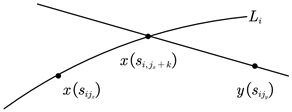﻿ 基于公路交通量的最佳乘车方案研究与设计

# 基于公路交通量的最佳乘车方案研究与设计Research and Design of the Best Ride Scheme Based on Road Traffic Volume

Abstract: This article mainly studies the problem of the bus ride schemes in a certain city. For riding schemes, we take the least transfer as the main goal, and the minimum time as the second goal, combined with the impact of highway traffic flow on road transit time—BPR (Bureau of Public Road) function, then select the optimal riding scheme that meets the requirements; for the choice of the strategy, we use the method of searching for bus routes, looking for the collection of bus routes passing through the starting point and ending point respectively. Then according to the direct, one-time transfer and two transfers, the two sets are searched separately, and the feasible plans are calcu-lated and the time spent on each plan is compared, so as to select the best riding scheme. Finally, we take Changsha City, Hunan Province as an example, combined with road traffic flow at different time periods to calculate the optimal riding scheme for 5 groups of travel routes.

1. 引言

2. 问题分析

3. 模型建立与求解

1) 每条公交线路上相邻公交车站的自由行驶时间相同，为 ${t}_{0}=4\mathrm{min}$

2) 两辆公交车的平均换乘时间为 ${T}_{0}=6\mathrm{min}$

3) 公交车的实际路段通行时间只与当前路段的通行量有关；

4) 将所有的公交线路视为单向的，即使是双向也视为两条单向，且无环行线。

${t}_{ij}={t}_{0}×\left(1+\alpha {\left(\frac{{Q}_{ij}}{{C}_{ij}}\right)}^{\beta }\right)$

3.1. 直达车算法

${A}_{x}\cap {A}_{y}\ne \varnothing$，则说明节点 $x$$y$ 之间存在直达线路，对 $\forall {L}_{i}\in {A}_{x}\cap {A}_{y}$，找出节点 $x$$y$ 在线路 ${L}_{i}$ 中相对应的节点是 ${s}_{i{j}_{x}}$${s}_{i{j}_{y}}$，如图1Figure 1. Map of through bus

${j}_{x}>{j}_{y}$，说明是反方向的线路，此时线路 ${L}_{i}$ 不可取；若 ${j}_{x}<{j}_{y}$，此时线路 ${L}_{i}$ 就是可行的线路，并计算乘坐此线路需要花费的时间

${t}_{i}=\underset{j={j}_{x}}{\overset{{j}_{y}}{\sum }}{t}_{ij}={t}_{0}×\underset{j={j}_{x}}{\overset{{j}_{y}}{\sum }}\left(1+\alpha {\left(\frac{{Q}_{ij}}{{C}_{ij}}\right)}^{\beta }\right)$

3.2. 一次换乘算法

${A}_{x}\cap {A}_{y}=\varnothing$，则说明节点 $x$$y$ 之间不存在直达线路，此时考虑一次换乘的方案， $\forall {L}_{i}\in {A}_{x}$，找出 $x$${L}_{i}$ 中对应的节点是 ${s}_{i{j}_{x}}$，对于经过 ${s}_{i{j}_{x}}$ 之后的节点 ${s}_{i,{j}_{x}+1},{s}_{i,{j}_{x}+2},\cdots$ 的线路集合 ${B}_{x}$，即

${B}_{x}=\underset{k}{\cup }{A}_{{s}_{i,{j}_{x+k}}}$

${B}_{x}\cap {A}_{y}\ne \varnothing$，说明此时存在一次换乘的方案，如图2，按照上述的直达车算法寻找可行的公交线路，同时计算相应趁车花费的时间加上换乘需要的时间即可得出总花费的时间，再对可行方案的集合进行比较进一步确定花费时间最少的方案。Figure 2. Map of public traffic transfer

3.3. 两次换乘算法

${B}_{x}\cap {A}_{y}=\varnothing$，说明此时不存在一次换乘的方案，此时考虑两次换乘， $\forall {L}_{i}\in {A}_{y}$，找出 $y$${L}_{i}$ 中对应的节点是 ${s}_{i{j}_{y}}$，对于经过 ${s}_{i{j}_{x}}$ 之前的节点 ${s}_{i,1},{s}_{i,2},\cdots ,{s}_{i,{j}_{y}}$ 的线路集合 ${B}_{y}$，即

${B}_{y}=\underset{k=1}{\overset{{j}_{y}}{\cup }}{A}_{{s}_{i,k}}$

${B}_{x}\cap {B}_{y}\ne \varnothing$，说明存在换乘两次的方案，同样按照直达车的算法找出相应的乘车路线并同时计算相应趁车花费的时间加上两次换乘需要的时间即可得出总花费的时间，再对可行方案的集合进行比较进一步确定花费时间最少的方案。

4. 实际应用——以湖南省长沙市公交线路为例Table 1. Optimal scheme of one public traffic transferTable 2. Optimal scheme of two public traffic transfer

2020年湖南省普通高等学校教学改革研究项目“数学与应用数学专业‘二三三四’人才培养模式的研究与实践”(编号：HNJG-2020-0276)。长沙理工大学大学生创新创业训练计划项目。

 古凌岚. GIS最短路径分析中Dijkstra算法的优化[J]. 计算机与数字工程, 2006, 34(12): 53-56.

 王炜, 张桂红. 城市道路路阻函数研究[J]. 重庆交通大学学报(自然科学版), 1992, 11(3): 84-92.

Top